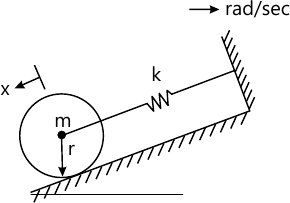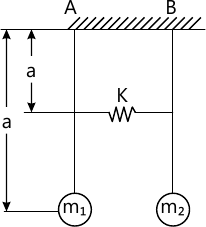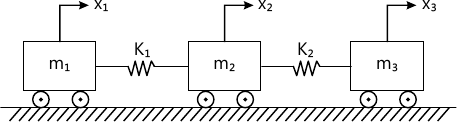MORE IN Dynamics of Machinery
SPPU Mechanical Engineering (Semester 7)
Dynamics of Machinery
May 2014
Total marks: --
Total time: --
INSTRUCTIONS
(1) Assume appropriate data and state your reasons
(2) Marks are given to the right of every question
(3) Draw neat diagrams wherever necessary

Answer any one question from Q1 and Q2
1 (a) Why single cylinder engines can not be balanced completely? Explain.
4 M
1 (b) Four masses A, B, C and D carried by a rotating shaft at radii 80 mm, 100 mm, 160 mm and 120 mm respectively are completely balanced. Masses B, C and D are 8 kg, 4 kg and 3 kg respectively. Determine the mass A and the relative angular positions of the four masses, if the planes are spaced 500 mm apart.
12 M

2 (a) Explain the method of direct and reverse crank to determine the unbalance forces in radial engines.
4 M
2 (b) The successive cranks of a 5 cylinder inline engine are at 144° apart. The spacing between the cylinder center lines is 400 mm. The length of crank and connecting rod are 100 mm and 450 mm respectively and the reciprocating mass for each cylinder is 20 kg. The engine speed is 630 RPM. Determine the maximum values of the primary and secondary forces and couples and the position of the central crank at which these occur.
12 M

Answer any one question from Q3 and Q4
3 (a) Define the following terms with respect to free damped vibrations:
i) Logarithmic decrement
ii) Damping coefficient
iii) Coulomb damping
iv) Damping factor.
4 M
3 (b) A homogeneous solid cylinder of mass ?m? is linked by a spring of constant 'k' N/m as shown in following fig. If it rolls without slipping, show that frequency of oscillations is.6 M
3 (c) An under damped shock absorber is to be designed for an automobile. It is required that initial amplitude to be reduced to 1/6th in one cycle. The mass of the automobile is 200 kg and damped period of vibration is one sec. Find the necessary stiffness and damping constant of shock absorber.
8 M

4 (a) Derive a relation to determine the loss of amplitude per cycle in case of Coulomb damping.
6 M
4 (b) A spring mass system with mass 'm' kg and stiffness 'k' N/m has a natural frequency of 'f' Hz. Determine the value of stiffness k1' of another spring which when arranged in conjunction with spring of stiffness 'k' in series will lower the natural frequency by 20%.
6 M
4 (c) A 500 kg vehicle is mounted on springs such that its static deflection is 1.5 mm. What is the damping coefficient of viscous damper to be added to the system in parallel with the springs, such that the system is critically damped?
6 M

Answer any one question from Q5 and Q6
5 (a) Derive an expression for magnification factor in case of steady state vibration subjected to an external periodic force F0sin (ωt).
8 M
5 (b) The springs of an automobile trailer are compressed 0.1 mm under its own weight. Find the critical speed when the trailer is passing over a road with a profile of sine wave whose amplitude is 80 mm and the wavelength is 14 m. Find the amplitude of vibration at a speed of 60 km/hr.
8 M

6 (a) The weight of an electric motor is 125 N and it runs at 1500 RPM. The armature weighs 35 N and its CG lies 0.05 cm from the axis of rotation. The motor is mounted on 5 springs of negligible damping so that the force transmitted is 1/11th of the impressed force. Assume that the weight of the motor is equally distributed among the 5 springs.
Determine
i) Stiffness of each spring
ii) Dynamic force transmitted to the base at operating speed.
iii) Natural frequency of the system.
8 M
6 (b) A vertical single stage air compressor having a mass 500 kg is mounted on spring having stiffness of 1.96×105 N/m and ξ=0.20. The rotating parts are completely balanced and the equivalent reciprocating parts weight 20kg. The strokes is 0.2m. Determine the dynamic amplitude of vertical motion and the phase difference between the motion and excitation force, if the compressor is operated at 200 RPM.
8 M

Answer any one question from Q7 and Q8
7 (a) An electric motor is driving a rotor through a single reduction gear. The particulars are as follows:
Gear ratio =4.5, M.I. of armature = 16 kg m2, M.I. of rotor = 40 kg-m2, Length of pinion shaft = 0.5 m, Diameter of pinion shaft = d1, Length rotor gear shaft = 3m, Diameter of rotor gear shaft=90mm.
Neglecting the inertia of gears, find the diameter 'd1' to have the node at the gear. Also find the natural frequency of the torsional vibrations and the amplitude of rotor vibration if the armature has amplitude of 17deg;, take G=80 GPa.
12 M
7 (b) What is the critical speed of the rotor without damping? Explain with the neat sketch.
6 M

8 (a) A double pendulum having length l is as shown in Fig. No. 2. Determine the natural frequencies of the pendulum when K = 50 N/M, m1 = 3 kg, l = 200 mm and a = 100 mm.10 M
8 (b) Determine the natural frequencies of the linear system as shown in Fig. 3 by Holzer method. Assume m1=m2=m3=1 kg and k1=k2=1 N/m.8 M

Answer any one question from Q9 and Q10
9 (a) Define sound fields. What are the various types of sound fields in the vicinity of a sound source?
6 M
9 (b) Show that when the distance from point of source is doubles the sound intensity level decreases by 6 dB.
6 M
9 (c) Determine the total sound power level due to five different sound pressure levels 95, 90, 92, 88 and 83 dB respectively.
4 M

10 (a) Explain different types of vibration exciters.
8 M
10 (b) A seismic instrument is used to find the displacement, velocity and acceleration of a machine running at 250 RPM. If the natural frequency of the instrument is 5 Hz and it records the displacement 5 mm. Determine displacement, velocity and acceleration of the vibrating machine. Assume no damping.
8 M

Answer any one question from Q11 and Q12
11 (a) Explain Piezoelectric accelerometer with neat sketch.
4 M
11 (b) What is microphone? Explain dynamic microphone with the sketch.
6 M
11 (c) What is FFT analyser? How the frequency spectrum is used to detect vibration related faults in a system.
6 M

Write short notes with neat sketches on any three:
12 (a) Vibration absorbers.
5 M
12 (b) Human hearing mechanism.
5 M
12 (c) Sound level meter.
5 M
12 (d) Different types of vibration isolators.
5 M
12 (e) Graphic level recorder and magnetic tape recorder.
5 M

More question papers from Dynamics of Machinery